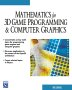# Maths - Matrix algebra - Eigenvectors and Eigenvalues

These quantities can have a geometric meaning and are also useful in matrix algebra, the geometric meaning is discussed on this page, it tells us something about the symmetry of a transform.

An eigenvector is a vector whose direction is not changed by the transform, it may be streached, but it still points in the same direction.

Each eigenvector has a corresponding eigenvalue which gives the scaling factor by which the transform scales the eigenvector. So the eigenvector is a vector and the eigenvalue is a scaler.

A given transform may have more than one eigenvector and eigenvalue pair depending on how many dimensions we are working in. For instance:

and so on.

As an example, if we have a rotation transform in 3 dimensions, then the eigenvector would be the axis of rotation since this is not altered by the transform and the corresponding eigenvalue would be +1 since the axis is not scaled by the rotation. If we have a rotation in 2 dimensions then the eigenvectors would be ±i where i is √-1 since all vectors in the plane change direction.

### Eigenvalues

The eigenvalues of a matrix [M] are the values of λ such that:

[M] v = λ v

where:

• v = eigenvector
• λ = lambda = eigenvalue

this gives:

|M - λ I| = 0

where I = identity matrix

this gives:

 m00-λ m01 m02 m10 m11-λ m12 m20 m21 m22-λ
= 0

so

(m00- λ) (m11- λ) (m22- λ) + m01 m12 m20 + m02 m10 m21 - (m00- λ) m12 m21 - m01 m10 (m22- λ) - m02 (m11- λ) m20 = 0

the values of λ are the eigenvalues of the matrix

### Eigenvectors

Associated with each eigenvalue λi is an eigenvector {ui} such that:

[M] {ui} = λi {ui}

where:

• [M] is a matrix
• λi is its eigenvalues (i=1,2,3)
• {ui}is its eigenvectors

## Program

There are a number of open source programs that can calculate eigenvalues and eigenvectors. I have used Axiom, how to install Axiom here.

To get a numeric solution for a given matrix, we can use eigenvalues(m) and eigenvectors(m) as shown here:

I have put user input in red:

 `(1) -> m := matrix[[1,4,7],[2,5,8],[3,6,9]]` ``` +1 4 7+ | | (1) |2 5 8| | | +3 6 9+ Type: Matrix Integer``` `(2) -> ev := eigenvalues(m)` ``` 2 (2) [0,%A | %A - 15%A - 18] Type: List Union(Fraction Polynomial Integer,SuchThat(Symbol, Polynomial Integer))``` `(3) -> eigenvectors(m)` ``` (3) + 1 + | | [[eigval= 0,eigmult= 1,eigvec= [|- 2|]], | | + 1 + +%G - 12+ |-------| | 6 | 2 | | [eigval= (%G | %G - 15%G - 18),eigmult= 1,eigvec= [|%G - 6 |]]] |------ | | 12 | | | + 1 + Type: List Record(eigval: Union(Fraction Polynomial Integer,SuchThat(Symbol,Polynomial Integer)),eigmult: NonNegativeInteger,eigvec: List Matrix Fraction Polynomial Integer) ```

Or we can find a general formula for a given matrix as shown here:

 `(1) -> msymb := matrix[[a,b,c],[d,e,f],[g,h,i]] ` ``` +a b c+ | | (1) |d e f| | | +g h i+ Type: Matrix Polynomial Integer ``` `(2) -> evsymb := eigenvalues(msymb)` ``` (2) [ %B | 2 ((a - %B)e - b d - %B a + %B )i + ((- a + %B)f + c d)h + 2 2 3 (b f - c e + %B c)g + (- %B a + %B )e + %B b d + %B a - %B ] Type: List Union(Fraction Polynomial Integer, SuchThat(Symbol,Polynomial Integer))``` `(3) -> eigenvectors(msymb)` ``` (3) [ [ eigval = %H | 2 ((a - %H)e - b d - %H a + %H )i + ((- a + %H)f + c d)h + 2 2 3 (b f - c e + %H c)g + (- %H a + %H )e + %H b d + %H a - %H , eigmult= 1, + 2 2 + | ((e - %H)h + b g)i - f h + (- c g - %H e + %H )h - %H b g | | ---------------------------------------------------------- | | 2 2 | | d h + (- e + a)g h - b g | | | eigvec= [| 2 2 |]] |(- d h + (- a + %H)g)i + (f g + %H d)h + c g + (%H a - %H )g| |-------------------------------------------------------------| | 2 2 | | d h + (- e + a)g h - b g | | | + 1 + ] Type: List Record(eigval: Union(Fraction Polynomial Integer, SuchThat(Symbol,Polynomial Integer)) ,eigmult: NonNegativeInteger,eigvec: List Matrix Fraction Polynomial Integer) ```

 metadata block see also: Correspondence about this page david Book Shop - Further reading. Where I can, I have put links to Amazon for books that are relevant to the subject, click on the appropriate country flag to get more details of the book or to buy it from them.Mathematics for 3D game Programming - Includes introduction to Vectors, Matrices, Transforms and Trigonometry. (But no euler angles or quaternions). Also includes ray tracing and some linear & rotational physics also collision detection (but not collision response). Other Math Books Specific to this page here:

This site may have errors. Don't use for critical systems.

Copyright (c) 1998-2021 Martin John Baker - All rights reserved - privacy policy.